Next: Intensity Rules Up: Time-Dependent Perturbation Theory Previous: Selection Rules

#Transitions in Hydrogen

Let us calculate the rate of spontaneous emission between the first excited state (i.e.,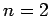) and the ground-state (i.e.,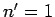) of a hydrogen atom. Now the ground-state is characterized by. Hence, in order to satisfy the selection rules (1149) and (1150), the excited state must have the quantum numbersand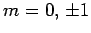. Thus, we are dealing with a spontaneous transition from a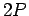to a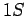state. Note, incidentally, that a spontaneous transition from a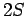to astate is forbidden by our selection rules.

According to Sect. 9.4, the wavefunction of a hydrogen atom takes the form(1151)

where the radial functionsare given in Sect. 9.4, and the spherical harmonicsare given in Sect. 8.7. Some straight-forward, but tedious, integration reveals that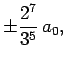(1152)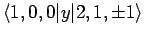(1153)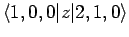(1154)

where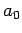is the Bohr radius specified in Eq. (679). All of the other possiblematrix elements are zero because of the selection rules. If follows from Eq. (1144) that the modulus squared of the dipole moment for thetransition takes the same value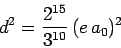(1155)

for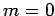,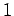, or. Clearly, the transition rate is independent of the quantum number. It turns out that this is a general result.

Now, the energy of the eigenstate of the hydrogen atom characterized by the quantum numbers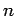,,is, where the ground-state energyis specified in Eq. (678). Hence, the energy of the photon emitted during atransition is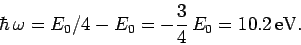(1156)

This corresponds to a wavelength of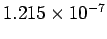m.

Finally, according to Eq. (1131), thetransition rate is written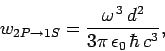(1157)

which reduces to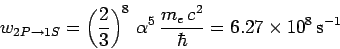(1158)

with the aid of Eqs. (1155) and (1156). Here,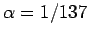is the fine-structure constant. Hence, the mean life-time of a hydrogenstate is(1159)

Incidentally, since thestate only has a finite life-time, it follows from the energy-time uncertainty relation that the energy of this state is uncertain by an amount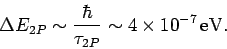(1160)

This uncertainty gives rise to a finite width of the spectral line associated with thetransition. This natural line-width is of order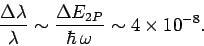(1161)Next: Intensity Rules Up: Time-Dependent Perturbation Theory Previous: Selection Rules
Richard Fitzpatrick 2010-07-20